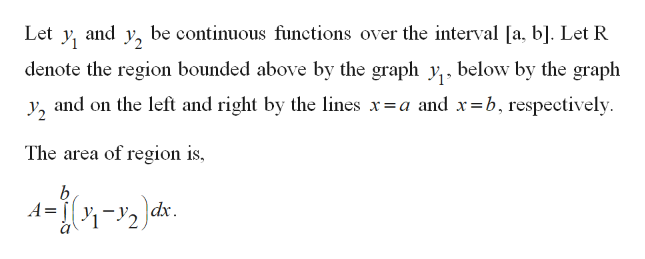# Find the area of the indicated region. Rounded to three decimal placesBetween y=ex and y=x for x in [0,2]

Question
2 views

Find the area of the indicated region. Rounded to three decimal places

Between y=ex and y=x for x in [0,2]

check_circle

Step 1

Find the area of bounded region.

Step 2

For finding Area betwee...help_outlineImage Transcriptioncloseand be continuous functions over the interval a, b]. Let R Let У 2 denote the region bounded above by the graph y below by the graph and on the left and right by the lines x=a and x=b, respectively У2 The area of region is b dx fullscreen

### Want to see the full answer?

See Solution

#### Want to see this answer and more?

Solutions are written by subject experts who are available 24/7. Questions are typically answered within 1 hour.*

See Solution
*Response times may vary by subject and question.
Tagged in

### Integration